Question

If a bond with a face value of \$1,000, 8 years to maturity, semiannual coupons, and the yield to maturity of 4% sells for \$1,067.89, what is its annual coupon rate? A. 4% B. 5% C. 2% D. 3% E. 2.5%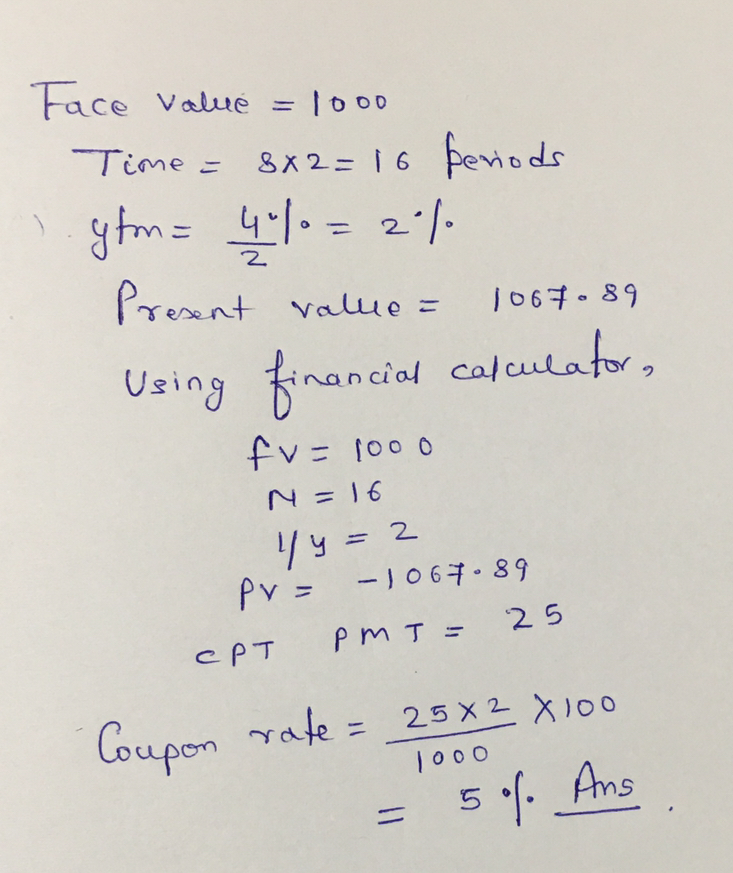#### Earn Coins

Coins can be redeemed for fabulous gifts.

Similar Homework Help Questions
• ### 25-year bond has a \$1,000 face value, a 10% yield to maturity, and an 8% annual...

25-year bond has a \$1,000 face value, a 10% yield to maturity, and an 8% annual coupon rate, paid semi-annually. What is the market value of the bond? Suppose a bond with a 10% coupon rate and semiannual coupons, has a face value of \$1000, 20 years to maturity and is selling for \$1197.93. What’s the YTM?

• ### The yield to maturity of a \$1,000 bond with a 7.4% coupon rate, semiannual coupons, and...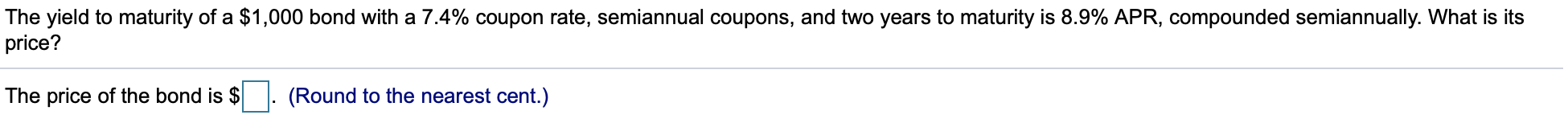The yield to maturity of a \$1,000 bond with a 7.4% coupon rate, semiannual coupons, and two years to maturity is 8.9% APR, compounded semiannually. What is its price? The price of the bond is \$ . (Round to the nearest cent.) Suppose a five-year, \$1,000 bond with annual coupons has a price of \$901.23 and a yield to maturity of 5.9%. What is the bond's coupon rate? The bond's coupon rate is %. (Round to three decimal places.)

• ### A T-bond with semi-annual coupons has a coupon rate of 3%, face value of \$1,000, and...

A T-bond with semi-annual coupons has a coupon rate of 3%, face value of \$1,000, and 2 years to maturity. If its yield to maturity is 4%, what is its Macaulay Duration? Answer in years, rounded to three decimal places

• ### A 30-year maturity bond with face value of \$1,000 makes semiannual coupon payments and has a...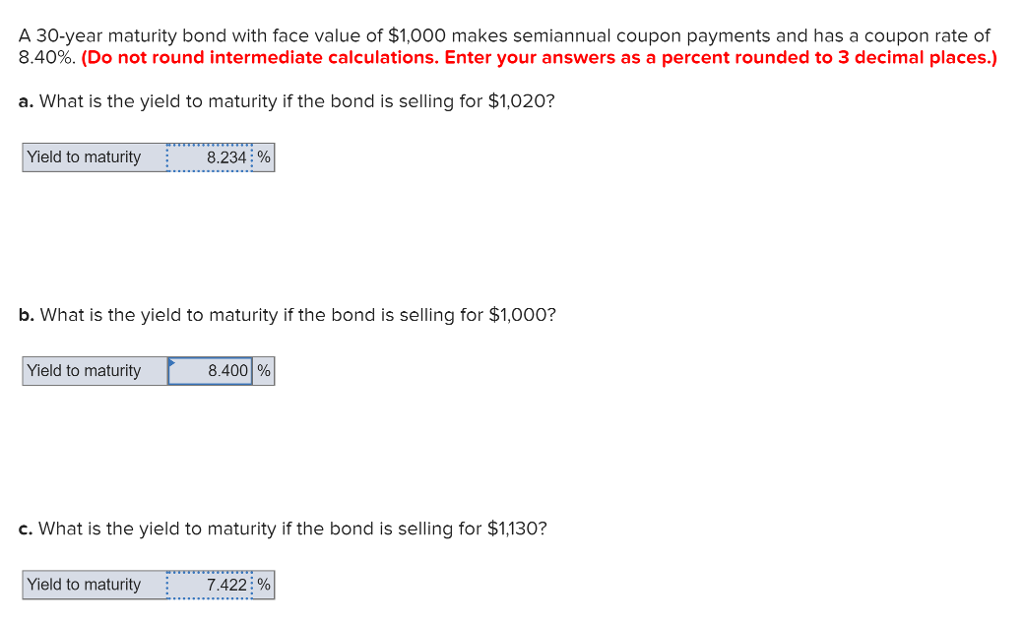A 30-year maturity bond with face value of \$1,000 makes semiannual coupon payments and has a coupon rate of 8.40%, (Do not round intermediat e calculations. Enter your answers as a percent rounded to 3 decimal places.) a. What is the yield to maturity if the bond is selling for \$1,020? Yield to maturity : 8234: % b. What is the yield to maturity if the bond is selling for \$1,000? Yield to maturity 8.4001 % c. What is the...

• ### Suppose a 10​-year, \$1,000 bond with a 8% coupon rate and semiannual coupons is trading for...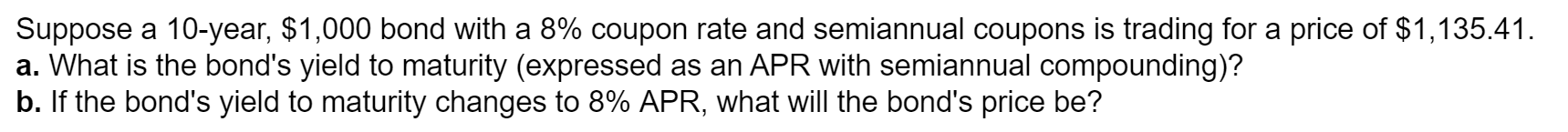Suppose a 10​-year, \$1,000 bond with a 8% coupon rate and semiannual coupons is trading for a price of \$1,135.41. a. What is the​ bond's yield to maturity​ (expressed as an APR with semiannual​ compounding)? b. If the​ bond's yield to maturity changes to 8% ​APR, what will the​ bond's price​ be? Suppose a 10-year, \$1,000 bond with a 8% coupon rate and semiannual coupons is trading for a price of \$1,135.41. a. What is the bond's yield to maturity...

• ### Bond Valuation A 20-year, 8% semiannual coupon bond with a par value of \$1,000 sells for...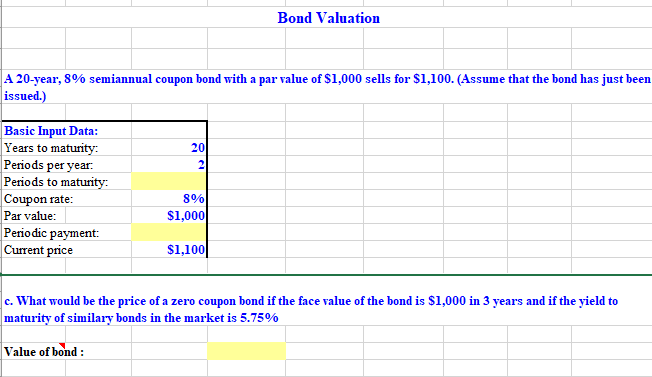Bond Valuation A 20-year, 8% semiannual coupon bond with a par value of \$1,000 sells for \$1,100. (Assume that the bond has just been issued.) 20 Basic Input Data: Years to maturity: Periods per year: Periods to maturity: Coupon rate: Par value: Periodic payment: Current price 8% \$1,000 \$1,100 c. What would be the price of a zero coupon bond if the face value of the bond is \$1,000 in 3 years and if the yield to maturity of similary...

• ### A 15-year maturity bond with face value of \$1,000 makes semiannual coupon payments and has a...

A 15-year maturity bond with face value of \$1,000 makes semiannual coupon payments and has a coupon rate of 6%. a. What is the bond’s yield to maturity if the bond is selling for \$1,110? Enter annual yield to maturity as your answer. (Do not round intermediate calculations. Round your answer to 3 decimal places.) Annual yield to maturity % b. What is the bond’s yield to maturity if the bond is selling for \$1,000? Enter annual yield to maturity...

• ### Bond Features Maturity (years) 5 Face Value = \$1,000 Coupon Rate = 3.00% Current Price =...

Bond Features Maturity (years) 5 Face Value = \$1,000 Coupon Rate = 3.00% Current Price = \$1,100 Coupon dates (Annual) Time to call (years) 3 Price if Called \$1,030.00 What is the bond's yield to call (YTC) (annual) if the bond is called at its first possible date? A. 0.62% B. 0.63% C. 2.75% D. -0.31% E. 2.73%

• ### A 30-year maturity bond with face value of \$1,000 makes semiannual coupon payments and has a...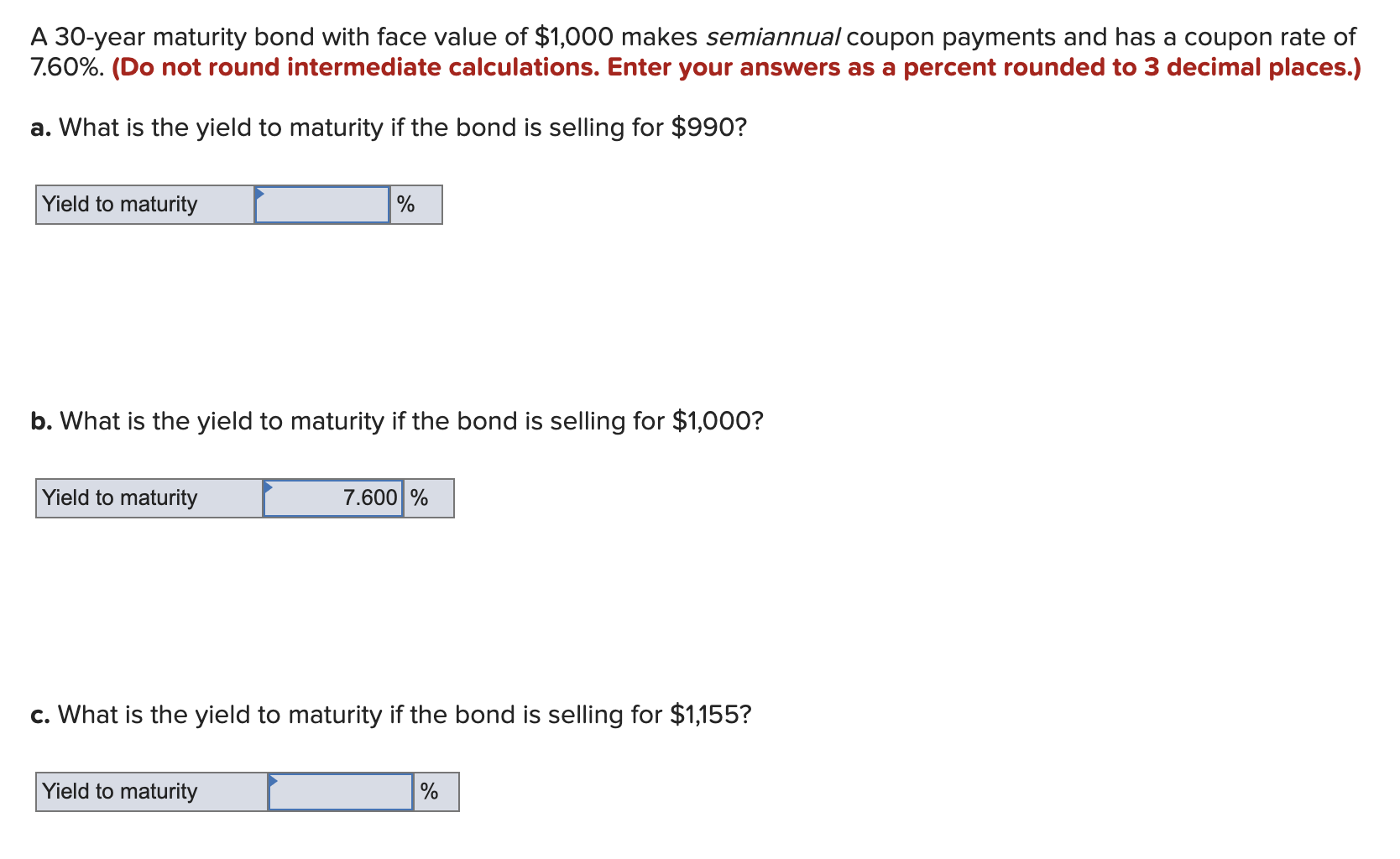A 30-year maturity bond with face value of \$1,000 makes semiannual coupon payments and has a coupon rate of 7.60%. (Do not round intermediate calculations. Enter your answers as a percent rounded to 3 decimal places.) a. What is the yield to maturity if the bond is selling for \$990? Yield to maturity Yield to maturity L % b. What is the yield to maturity if the bond is selling for \$1,000? Yield to maturity 7.600 % | c. What...

• ### What is the price of a 3 -year, 8.1% coupon rate, \$1,000 face value bond that...

What is the price of a 3 -year, 8.1% coupon rate, \$1,000 face value bond that pays interest quarterly if the yield to maturity on similar bonds is 11.9 % What is the yield to maturity of at 9.9% semiannual coupon bond with a face value of \$1,000 selling for \$894.71 that matures in 11 years? The annual yield to maturity of the bond is: (Select the best choice below.) A.11.62% B.11.039% C.12.201% D.13.131% E.9.528%